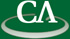## Eigenvalues, invariant factors and random integer matrices (with a little bit of computing)

### 3:00pm Monday December 14th, 2015, in TASC II Room 8500 (PIMS seminar room).

Mark Giesbrecht, University of Waterloo.

```Abstract
Integer matrices are typically characterized by the lattice of linear
combinations of their rows or columns. This is captured nicely by the
Smith canonical form, a diagonal matrix of "invariant factors", to
which any integer matrix can be transformed through left and right
multiplication by unimodular matrices.

But integer matrices can also be viewed as matrices of real numbers,
with eigenvalues and eigenvectors, and we all learn early on that every
real matrix is similar to a unique one in Jordan canonical form.

It would seem a priori that the invariant factors and the eigenvalues
would have little to do with each other.  Yet we will show that for
"almost all" matrices the invariant factors and the eigenvalues are
equal under a p-adic valuation.

All the methods are elementary and no particular background beyond
linear algebra will be assumed.  A much-hoped-for link and some open
problems on algorithms for sparse integer matrices will be explored.

This is joint work with Mustafa Elsheikh
```